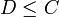# ZJ-subgroup contains D*-subgroup

This article gives the statement, and possibly proof, of the subgroup obtained from one subgroup-defining function, namely D*-subgroup, always being contained in the subgroup obtained from another subgroup-defining function, namely ZJ-subgroup.
View other such results

## Statement

Suppose$p$ is a prime number and$P$ is a finite p-group. Then, the ZJ-subgroup of$P$ contains the D*-subgroup of$P$.

## Definitions used

### D*-subgroup

Denote by$\mathcal{D}^*(P)$ the set:$\mathcal{D}^*(P) = \{ A \le P \mid A \mbox{ is abelian and }\operatorname{class}(\langle A,x \rangle ) \le 2 \implies x \in C_P(A) \ \forall \ x \in P \}$

### ZJ-subgroup

Denote by$\mathcal{A}(P)$ the set of abelian subgroups of maximum order in$P$. Then, the ZJ-subgroup$ZJ(P)$ is defined as the intersection of all members of$\mathcal{A}(P)$. Equivalently, it is the center of the join of all these subgroups (see join of abelian subgroups of maximum order).

## Proof

It suffices to show that$D$ is contained in every abelian subgroup of maximum order in$P$, because$ZJ(P)$ is the intersection of all these.

Given: A finite$p$-group$P$,$D = D^*(P)$,$B$ is an abelian subgroup of maximum order in$P$.

To prove:$D \le B$

Proof:

Step no. Assertion/construction Facts used Given data used Previous steps used Explanation
1$D$ is an abelian characteristic subgroup, hence abelian normal subgroup of$P$. Fact (1)$D$ is the D*-subgroup abelianness follows from containment in$\mathcal{D}^*(P)$, and characteristic follows from it being the unique maximal element.
2 The product$BD$ is a subgroup of$P$ that is metabelian. Step (1) [SHOW MORE]
3$B$ is an abelian subgroup of maximum order in$BD$.$B$ is abelian of maximum order in$P$ Given-direct
4 There exists a subgroup$C$ of$BD$ that is abelian and normalized by$D$, is contained in the normal closure$B^{BD}$, and has the same order as$B$ (and hence maximum order among abelian subgroups of$BD$). In particular,$D$ normalizes$C$. Fact (2) Apply Fact (2) in the ambient group$BD$.
5$CD$ has nilpotency class at most two. Fact (3) Steps (1), (4) Apply Fact (3) and Steps (1) and (4) inside the ambient group$CD$. Note here that$C$ is not necessarily normal, but since it is normalized by$D$, it is normal in the join$CD$, which is the group within which we apply Fact (3).
6 For any$x \in C$, the subgroup$\langle D , x \rangle$ is a subgroup of$P$ of class at most two. Fact (4) Step (5) Fact-step combination direct
7 For any$x \in C$,$x$ centralizes$D$.$D = D^*(P)$ and hence$D \in \mathcal{D}^*(P)$. Step (6) Apply Step (6) and the condition that$D \in \mathcal{D}^*(P)$ to get the result.
8 The product$CD$ is an abelian subgroup of$P$ Steps (1), (4), (7) By Steps (1), (4), both are abelian. By Step (7), they centralize each other. Hence their product is also abelian.
9$CD = C$, so$D \le C$. Steps (4), (8)$CD$ is a subgroup of$BD$ that contains$C$. By Step (4),$C$ is abelian of maximum order in$BD$. This forces$CD = C$.
10$D \le B^{BD} = B^D$. Steps (4), (9)$D \le C$, and by Step (4),$C \le B^{BD}$.
11$BD = B^{BD}$, so$B$ is a contranormal subgroup of$BD$. Step (10) By the previous step,$BD \le B^{BD}$. The other direction,$B^{BD} \le BD$, is definitional.
12$B = BD$, so$D \le B$. Fact (5) we are working with$p$-groups Step (11) Step-fact-given combination direct
This proof uses a tabular format for presentation. Provide feedback on tabular proof formats in a survey (opens in new window/tab) | Learn more about tabular proof formats|View all pages on facts with proofs in tabular format